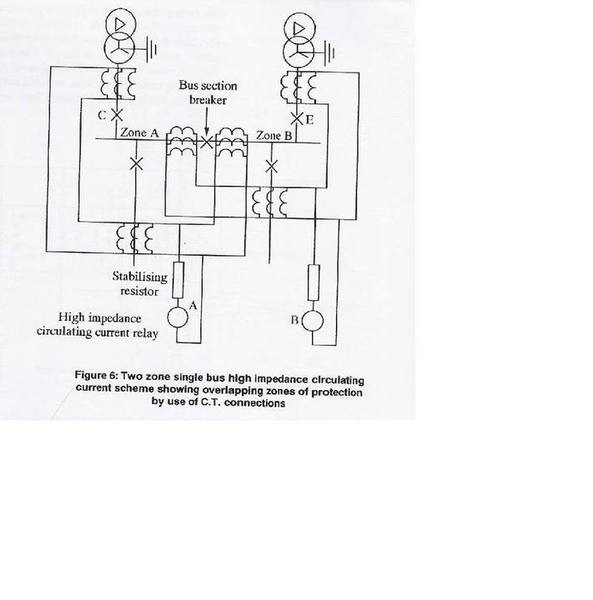# High impedance circulating current scheme(calculate stabilising resistor)

Hi,

I am going through some texts but it was not very clear.I hope some experts here can help me on this.

The max voltage across the relay and stabilising resistor is given by;

(If/N)(Rs+Rb)

where If - max short circuit rating, A
N - current transformer, CT ratio
Rs - CT internal resistance

Relay impedance at setting current is given by VA burden/(I setting)2

Total relay circuit impedance = V max/I setting

Stabilising resistance = total relay circuit impedance - relay impedance at setting current.

Now if I have Isc=2000A
CT ratio=200:5
Rs=0.6 ohm
Rb=0.4 ohm

Hence max voltage calculated from the equation above = 50V.

Now here is the problem to find relay impedance at setting current,

Do I assume the relay setting and the VA burden or if these two parameters can be calculated from the above information?

Thank you.

p/s: I dont think we need to look at the circuit diagram because it just shows the protection scheme without going anywhere related to the calculation.

Edit:Posted the circuit diagram of the high impedance circulating current scheme.Last edited:

This looks very much like an incomplete version of a homework or coursework problem, presented without the schematic diagram which originally accompanied the text.

Some readers may be sufficiently familiar with this material to determine the original question, but it would be much better if you would present the question clearly in its original form.

This looks very much like an incomplete version of a homework or coursework problem, presented without the schematic diagram which originally accompanied the text.

Some readers may be sufficiently familiar with this material to determine the original question, but it would be much better if you would present the question clearly in its original form.

Aww,man,this is not a homework.As you can see,all the equations are there.I can even provide the step by step answers.It just that the text plainly stated the VA burden as 1 VA and relay setting as 0.1A,which I dont understand where they came from.

The final answer would be 400 ohm for the stabilising resistor.

I just need the explaination and this is not homework.

Thank you.

berkeman
Mentor
Hi,

I am going through some texts but it was not very clear.I hope some experts here can help me on this.

The max voltage across the relay and stabilising resistor is given by;

(If/N)(Rs+Rb)

where If - max short circuit rating, A
N - current transformer, CT ratio
Rs - CT internal resistance

Relay impedance at setting current is given by VA burden/(I setting)2

Total relay circuit impedance = V max/I setting

Stabilising resistance = total relay circuit impedance - relay impedance at setting current.

Now if I have Isc=2000A
CT ratio=200:5
Rs=0.6 ohm
Rb=0.4 ohm

Hence max voltage calculated from the equation above = 50V.

Now here is the problem to find relay impedance at setting current,

Do I assume the relay setting and the VA burden or if these two parameters can be calculated from the above information?

Thank you.

p/s: I dont think we need to look at the circuit diagram because it just shows the protection scheme without going anywhere related to the calculation.

Why are you talking about a current transformer ratio for a relay? This is confusing. Posting the diagram would help a lot.

Why are you talking about a current transformer ratio for a relay? This is confusing. Posting the diagram would help a lot.

Ok,I have posted the circuit diagram.Thank you.

To Berkeman:- CT ratio needed as primary (not secondary) current given.

To All:- I have a software package developed that calculates stabilizing resistor values as well as much more, it's designed for HV Commissioning Engineers. If anyone's interested please send me a PM.

Last edited by a moderator:
Last edited by a moderator: